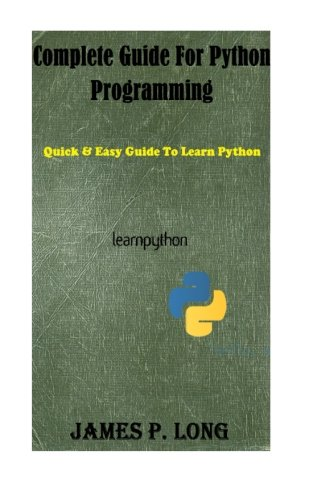# Complete Guide For Python Programming

Description

Complete Guide For Python Programming: Quick & Easy Guide To Learn Python

The Book is Quick and Easy Guide to learn Python Programming. This book includes all the basics of python, functions, classes, databases use in python programming. With this book you can learn professional Python style, best practices, and good programming habits. You can also improve Improve application performance by writing extensions using multithreading. You can become a good python programmer by going through this book. Book also contains basic programs written in python. There are around 50 programs you will find in this book. In this bookyou will find: Python Versions Some Commonly used Operations in Python Python Interactive Python Implemetations Python Compilers & Numerical Accelerators Logical And Physical Line in Python Python Indentation Python Standard Library Creating Classes & Objects Documenting Your Code Python – Object Oriented Programming Python Database Classes Methods Instances Python Database Access Python Networking Sending Mail in Python

1. Introduction
2. Python Versions
3. Some Commonly used Operations in Python
4. Printf Format Strings
5. Python Interactive – Using Python As A Calculator
6. Python Implementations
7. Python Compilers & Numerical Accelerators
8. Logical And Physical Line in Python
9. Python Indentation
10. Python Standard Library
11. Creating Classes & Objects
13. Python – Object Oriented Programming
14. Python Database
15. Classes
16. Methods
17. Instances
18. Python Database Access
19. Python Networking
20. Sending Mail in Python
22. Python xml processing
23. Python Programs
24. Python Program to Add Two Matrices
25. Python Program to Add Two Numbers
26. Python Program to Calculate the Area of a Triangle
27. Python Program to Check Armstrong Number
28. Python Program to Check if a Number is Odd or Even
29. Python Program to Check if a Number is Positive, Negative or Zero
30. Python Program to Check if a String is Palindrome or Not
31. Python Program to Check Leap Year
32. Python Program to Check Prime Number
33. Python Program to Convert Celsius To Fahrenheit
34. Python Program to Convert Decimal into Binary, Octal and Hexadecimal
35. Python Program to Convert Decimal to Binary Using Recursion
36. Python Program to Convert Kilometers to Miles
37. Python Program to Count the Number of Each Vowel
38. Python Program to Display Calendar
39. Python Program to Display Fibonacci Sequence Using Recursion
40. Python Program To Display Powers of 2 Using Anonymous Function
41. Python Program to Display the multiplication Table
42. Python Program to Find Armstrong Number in an Interval
43. Python Program to Find ASCII Value of Character
44. Python Program to Find Factorial of Number Using Recursion
45. Python Program to Find Factors of Number
46. Python Program to Find Hash of File
47. Python Program to Find HCF or GCD
48. Python Program to Find LCM
49. Python Program to Find Numbers Divisible by Another Number
50. Python Program to Find Sum of Natural Numbers Using Recursion